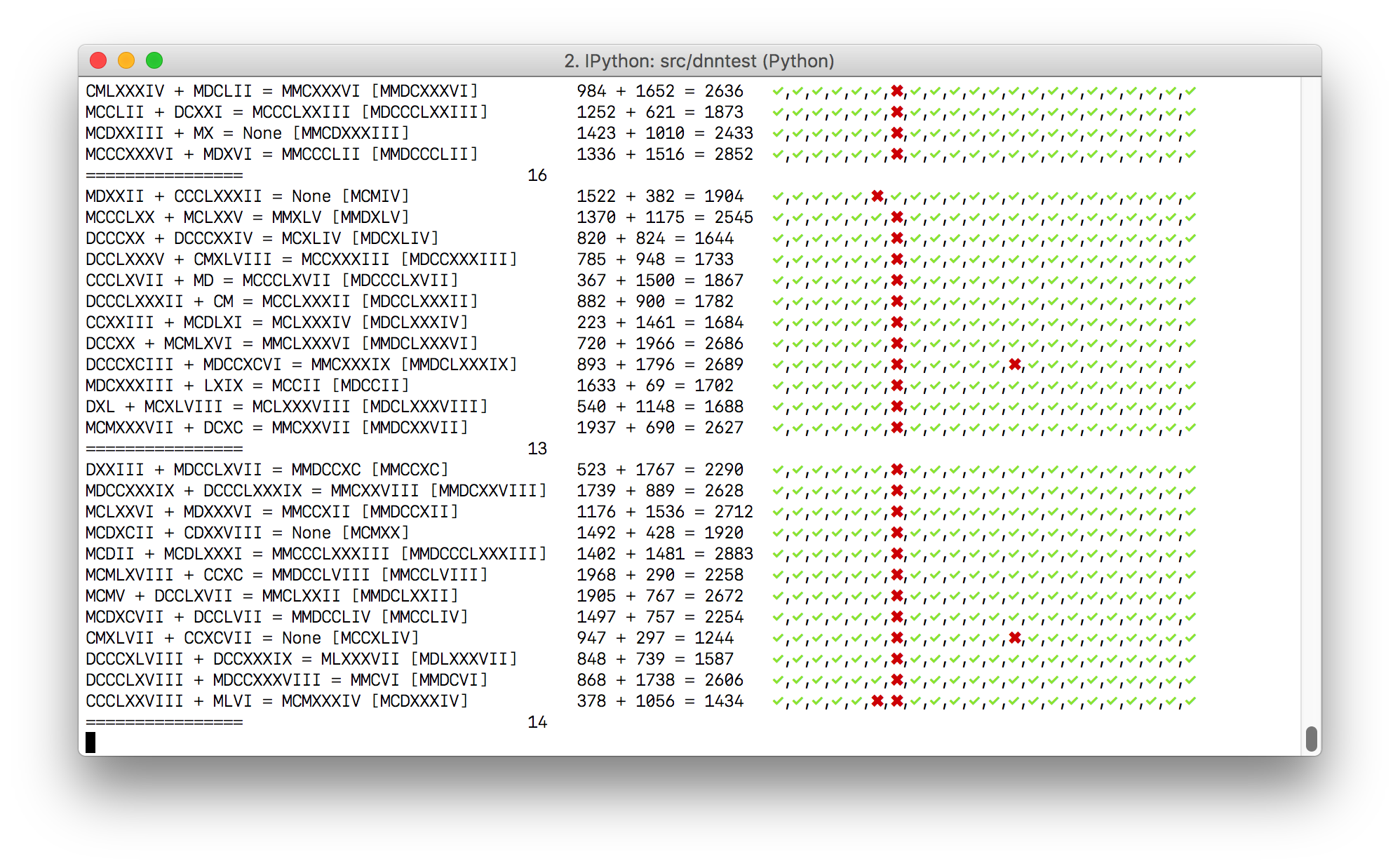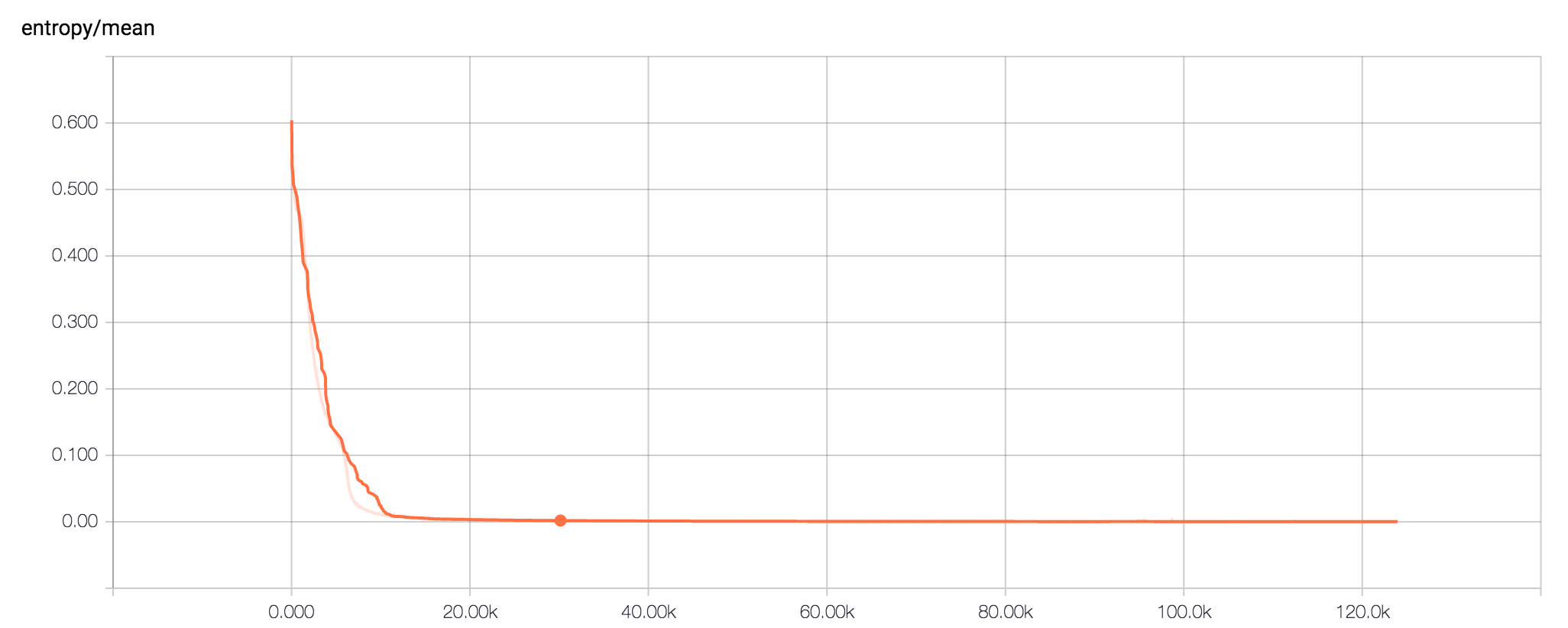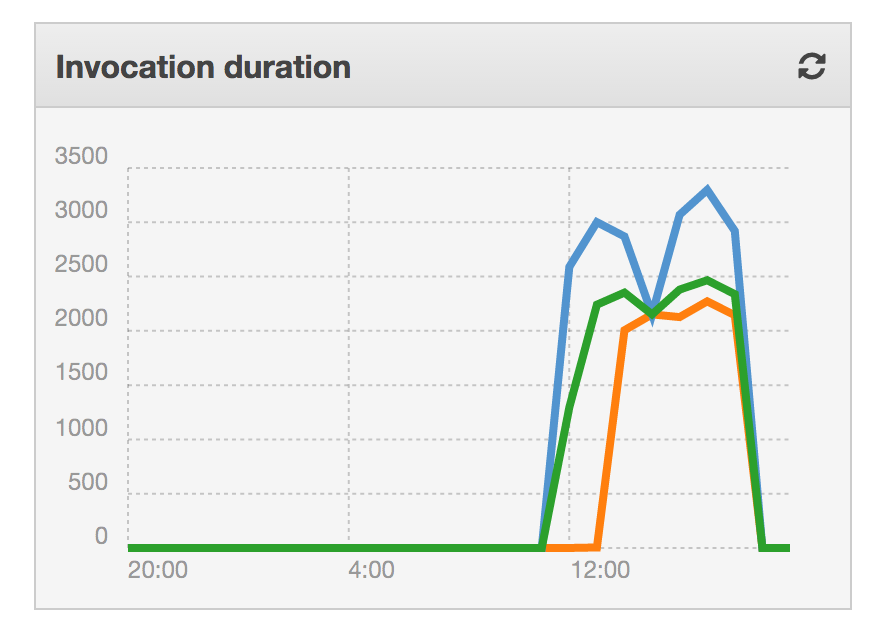# Roman Numeral Calculator

The network has only been trained on numbers up to 2000.
Numbers above this (up to 4000) will give funky results
+
=

# How to Use

Either:

• type any number (up to 2000) into the top boxes
• or type a valid roman numeral (up to MM) into the boxes just underneath.

As you type, the sequence of 0s below will automatically update, adding 1s to represent each numeral.

Pressing Go sends these sequences of 0s and 1s to an amazon Lambda function that runs a neural network on the inputs to calculate the result of adding these numbers.

The result is returned as a sequence of 1s and 0s like the inputs, the javascript on this page then maps the 1s back onto numerals, and displays the result in the boxes below the button

All conversion to «-» from decimal numbers is done in javascript on this page, the lambda function performs the calculation just using sets of 1s and 0s.

# How it works

The neural network is built/trained using TensorFlow. Each numeral is encoded using a fixed template such that the funky subtraction rules (IV = 5 - 1) can be represented unambiguously: MMMMCMDCCCXCLXXXIXVIII

Positions are present for each numeral, with different positions for where a numeral is subtracting from a larger value. For example, looking at the numbers 0-9:

DecimalNumeralTemplate
I X V I I I
0_000000
1I000001
2II000011
3III000111
4IV101000
5V001000
6VI001001
7VII001011
8VIII001111
9IX110000

This format allows tensorflow to very naturally read the list of 0s and 1s as a number vector, and treat it as any other input vector.

The two numbers are converted to this format, and then just concatenated into one list. For example, 1999 + 1234 becomes MCMXCIX + MCCXXXIV which becomes 0001110000110000110000 + 0001000011000111101000 (using above rules) which ends up as vector of:

[0,0,0,1,1,1,0,0,0,0,1,1,0,0,0,0,1,1,0,0,0,0,0,0,0,1,0,0,0,0,1,1,0,0,0,1,1,1,1,0,1,0,0,0]

This vector is fed into tensorflow, which runs the network, and produces a vector of confidences that each output value should be 1 or 0 depending on the input. (high values mean very sure that the output should be 1, low values mean very sure the output should be 0.

In this example, the result is (rounded):

[-42, 27, 121, 172, -95, -87, -27, -56, 15, 62, -54, -38,-37, 9, 38, 52, -10, -18, -7, 19, 17, 16]

The net is really sure that the 3rd position should be 1 (confidence of 121) whereas the 14th position is much less clear (confidence of only 9).

To turn theses confidences into readable output, values > 0.5 are interpreted as '1', while values < 0.5 are turned into '0', in this case (the threshold of 0.5 is a legacy from when the code was using normalized, positive only outputs:

[0,1,1,1,0,0,0,0,1,1,0,0,0,1,1,1,0,0,0,1,1,1]

The template MMMMCMDCCCXCLXXXIXVIII is then directly mapped to this list. Characters in the template are included if the corresponding vector value is 1, or skipped if the value is 0

[0,1,1,1,0,0,0,0,1,1,0,0,0,1,1,1,0,0,0,1,1,1] [M M M M C M D C C C X C L X X X I X V I I I]

Giving the correct result: MMMCCXXXIII

## The Network

Each number encodes to 22 values (either 1 or 0). We're adding two numbers so the input is a set of 44 numbers, as a numpy array of float32s.

To let the network learn all the rules, it has 4 layers of neurons: 2 layers with 66 nodes, 1 layer with 44 nodes, and an output layer producing 22 outputs. This is more than should strictly be required, but training was fast enough(given how easy getting training data is) with this net, so I left it.

Training this sort of toy graph is very easy, as the inputs and expected outputs can be generated by the computer already, training the network on millions of datapoints is trivial. The training script trained in batches of 500, with a test round of 50 numbers every 50 batches.

I wrote a function to show the results of each training round in the console. For each item in the training set that didn't match, it printed out info showing the numeral and decimal inputs/expected outputs, and a per-output tick or cross to say if that particular output bit was correct or notAfter a period of time, certain 'troublesome' bits in the output become apparent as a streak of red down the screen.

Also, by always printing a line of separator characters, and only printing test output where it didn't match the expected value, it became trivial to see when the net was trained, by seeing how many uninterrupted lines of separators were output.

The neural network was configured to use ELU (Exponential Linear Units) activation function, unlike the more common relu function, this allows neurons to negatively affect the output, something that helps with this weird usage of neural nets.

Often, nets are used to do distinct classification: the animal is either a dog or a cat, but never both. In this case, for a given input, many output should be active at the same time (we have output that are made with than 1 numeral), so the correct entropy calculation had to be chosen. I used sigmoid_cross_entropy_with_logits here, as this supports 'multilabel' classification.

I played around with different optimizers, and learning rates. In this situation, there is no noise in the data, unlike, for example, pictures of animals, where the connection between contributing features and classification is very weak, in this case, every bit of input is relevant to the correct output, and always accurate (no mis-classifications, computers are remarkably good at not making mistakes when adding numbers). In the end, the AdamOptimizer came out as a good option, with a learning rate of 0.7.

To give an idea of the learning rate, I ran a training set with summaries turned on, to view the results in tensorboard. Each batch was 5,000 samples. After 125,000 batches (600,000,000 samples!) The resulting cross-entropy was around 2 x 10-4, gibing a 0.2% error rate.## Amazon Lambda

This code seemed a great candidate for a Lambda function. Self-contained, single functionality service, hosted on the web with no changing state.

Setting it up, and getting the code running, was very straight-forward. Amazon provides a vanilla python 2.7 environment for running the lambda, and allows uploading a .zip file with all the extension modules required on top of that.

This script requires tensorflow and numpy to work, so I fired up a standard ec2 instance (Amazon Linux AMI), copied in my script (called main.py) and ran the following:

#!/bin/bash set -ex SCRIPT_FILE="main.py" S3_BUCKET=<S3 BUCKET NAME> # Remember to set AWS_ACCESS_KEY_ID and AWS_SECRET_ACCESS_KEY environment variables sudo yum groupinstall -y "Development Tools" ZIP_PATH=\$PWD/payload.zip virtualenv build ( . build/bin/activate pip install -r requirements.txt touch build/lib/python2.7/site-packages/google/__init__.py (cd build/lib/python2.7/site-packages && zip -r \$ZIP_PATH . -x \*.pyc) (cd build/lib64/python2.7/site-packages && zip -r \$ZIP_PATH . -x \*.pyc) ) zip \$ZIP_PATH net* # the saved network weights zip \$ZIP_PATH \$SCRIPT_FILE s3put -b \$S3_BUCKET -p \$PWD payload.zip

This script:

1. installs the dependencies (listed in requirements.txt) into a new virtual environment
2. zips up all the libraries
3. Adds my main.py file to the zip
4. Uploads the zip to s3

The lambda web UI makes it very simple to import a file into a lambda function from S3. Once uploaded, the Handler (under Configuration) has to be set to the module and function name to be run (in this case: main.main - a function called main in the main.py file)

Finally, the lambda function has to be configured with an API gateway endpoint to make it available over HTTP, and all should be fine.Initially, I had all of the tensorflow setup and run code in the main function, this lead to the lambda function running very slowly (and expensively). The API was taking ~3 seconds to return each time.

To work around this, I re-wrote the script to configure the graph, load the stored net data, and enter a session at import time, then in the handler function, it just evaluates the graph with the passed in data. This reduced the query time to about 200ms (>10x faster).